MathsNext: What polynomial Up: Fast CRC32 in Software Previous: Introduction

Maths

Thebits of data that are the message to be checked by the CRC can be represented by a polynomial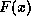of degree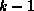. As an example the one octet message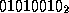is represented by the polynomial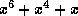. In general, leading zero bits do not affectwhereas trailing zero bits each add a factor of. The generating polynomial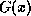is chosen by very clever mathematicians and is essentially fixed. The polynomial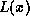is used to represent 32 successive ones.

The CRC is defined as the ones complement of a remainder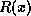obtained from the modulo two division of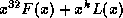by the generating polynomial. I.e. the CRC is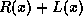.

The multiplication by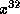corresponds to leaving space at the end of the message for the generated CRC. The addition of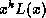(which is equivalent to inverting the first 32 bits of the message) precludes erroneous addition or deletion of leading zeros. The inversion of the CRC before sending it precludes erroneous addition of trailing zeros.

Transmission

As described: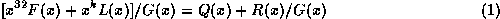for some quotient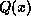and remainder. The CRC is the complement.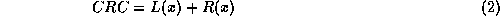The transmitted message denoted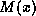isfollowed by the CRC.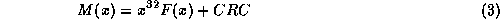Reception

At the receiver the operation is again repeated. This time however the value used israther thanalso the message is 32 bits longer sois replaced by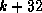. We consider: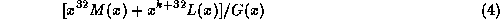Factoring outwe get: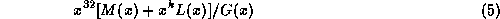Substituting (3) and thence (2) gives: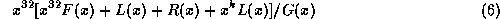Taking the divisor inside we can now substitute (1).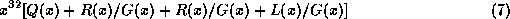From which theterms cancel in our modulo two arithmetic. We are, or course, only interested in the remainder of the division which is therefore.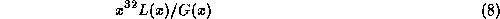Which is a known constant value (called the residual or residue)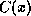for all messages.Next: What polynomial Up: Fast CRC32 in Software Previous: Introduction

Richard Black